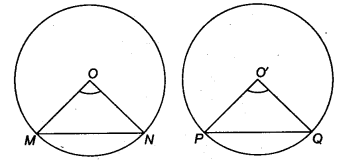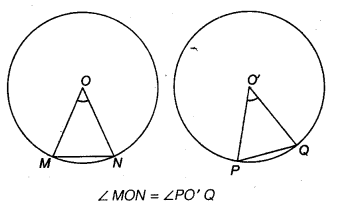## NCERT Solutions for Class 9 Maths Chapter 11 Circles Ex 11.2

NCERT Solutions for Class 9 Maths Chapter 11 Circles Ex 11.2 are part of NCERT Solutions for Class 9 Maths. Here we have given NCERT Solutions for Class 9 Maths Chapter 11 Circles Ex 11.2.

 Board CBSE Textbook NCERT Class Class 9 Subject Maths Chapter Chapter 11 Chapter Name Circles Exercise Ex 11.2 Number of Questions Solved 2 Category NCERT Solutions

## NCERT Solutions for Class 9 Maths Chapter 11 Circles Ex 11.2

Question 1.
Recall that two circles are congruent, if they have the same radii. Prove that equal chords of congruent circles subtend equal angles at their centres
Solution:
Given MN and PQ are two equal chords of two congruent circles with centre at O and O’.
To prove ∠ MON = ∠ PO’QProof In ∆ MON and ∆ PO’Q, we have
MO = PO’ (Radii of congruent circles)
NO = QO’ (Radii of congruent circles)
and MN = PQ (Given)
∴ By SSS criterion, we get
∆ MON = ∆ PO’Q
Hence, ∠ MON = ∠ PO’Q (By CPCT)

Question 2.
Prove that, if chords of congruent circles subtend equal angles at their centres, then the chords are equal.
Solution:
Given MN and PQ are two chords of congruent circles such that angles subtended by .
these chords at the centres O and O’ of the circles are equal.To prove MN = PQ
Proof In ∆ MON and ∆ PO’Q, we get
MO = PO’ (Radii of congruent circles)
NO = QO’ (Radii of congruent circles)
and ∠MON = ∠PO’Q (Given)
∴ By SAS criteria, we get
∆ MON = ∆ PO’Q
Hence, MN = PQ (By CPCT)

We hope the NCERT Solutions for Class 9 Maths Chapter 11 Circles Ex 11.2, help you. If you have any query regarding NCERT Solutions for Class 9 Maths Chapter 11 Circles Ex 11.2, drop a comment below and we will get back to you at the earliest.